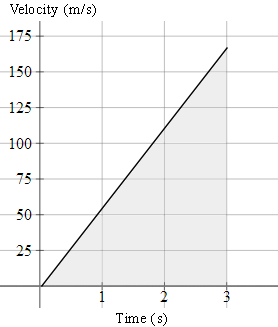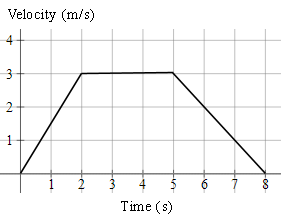Search IntMath
Close

450+ Math Lessons written by Math Professors and Teachers

5 Million+ Students Helped Each Year

1200+ Articles Written by Math Educators and Enthusiasts

Simplifying and Teaching Math for Over 23 Years

# 2. Acceleration (v-t) Graphs

by M. Bourne

Acceleration is the change in velocity per time.

A common unit for acceleration is "ms"^-2. An acceleration of 7\ "ms"^-2 means that in each second, the velocity increases by 7\ "ms"^-1 (also written as 7\ "m/s").

We can find the acceleration by using the expression:

text(acceleration)=text(change in velocity)/text(change in time

We can write the above using the equivalent

text(acceleration)=(Deltav)/(Deltat

where the Greek letter Δ (Delta) means "change in".

In other words, the slope of the velocity graph tells us the acceleration.

## The Area Under the v-t Graph

A very useful aspect of these graphs is that the area under the v-t graph tells us the distance travelled during the motion.

This concept is important when we find areas under curves later in the integration chapter.

### Example 1

A particle in a generator is accelerated from rest at the rate of 55\ "ms"^-2.

a. What is the velocity at t = 3\ "s"?

b. What is the acceleration at t = 3\ "s"?

c. What is the distance travelled in 3 seconds?

d. Graph the acceleration (as a v - t graph) for 0 ≤ t ≤ 3\ "s".

a. Velocity = 55 × 3 = 165\ "ms"^-1

b. The acceleration is a constant 55\ "ms"^-2, so at t = 3\ "s", the acceleration will be 55\ "ms"^-2.

c. The distance travelled in 3 seconds is 165 × 1.5 = 247.5\ "m". We obtain this from the area under the line between 0 and 3 (i.e. the area of the shaded triangle below).

d. Note in the graph that we have velocity on the vertical axis, and the units are m/s.

The graph finishes at (3, 165).### Recall: Area of a trapezoid

NOTE: A trapezoid has one pair of parallel sides. (It's called a "trapezium" in British English.)

A trapezoid (trapezium), parallel sides a and b, height h.

We find the area of a trapezoid by simply finding the average of the two parallel sides, and multiplying by the distance between them (usually called the "height"):

text(Area)=((a+b)h)/2

We'll make use of this result in the next question.

### Example 2

A body moves as described by the following v-t graph.a) Describe the motion.

b) What is the distance travelled during the motion?

c) What is the average speed for the motion?

a) From t = 0 to 2, the acceleration was a=(Deltav)/(Deltat)=3/2=1.5\ text(ms)^-2

From t = 2 to 5, the acceleration was 0\ "ms"^-1.

The body was neither speeding up nor slowing down.

From t = 5 to 8, the acceleration was a=(Deltav)/(Deltat)=(-3)/3=-1\ text(ms)^-2

The body was slowing down, so the acceleration was negative.

b) The distance travelled is the area of the trapezoid (trapezium).

text(distance)=((a+b)h)/2

=((8+3)(3))/2

=16.5\ text(m)

c) text(average speed)=text(distance travelled)/text(time taken)

=16.5/8

=2.1\ text(ms)^-1

## Problem SolverThis tool combines the power of mathematical computation engine that excels at solving mathematical formulas with the power of GPT large language models to parse and generate natural language. This creates math problem solver thats more accurate than ChatGPT, more flexible than a calculator, and faster answers than a human tutor. Learn More.